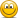Indicator function - Expected shortfall

emilioalzamora1

Well-Known Member
Hi @David Harper CFA FRM,

Perhaps this is a question for the broad community and I would like to ask sth. very specific about the indicator function on p.66 in Dowd's book "Measuring market risk"

He writes that "the ES gives all tail-loss quantiles an equal weight, and other quantiles aweight of 0. Thus the ES is a special case of Mφ obtained by setting φ(p) to the following..."

And then he defines the weighting or so-called indicator function as:

* 0 if p < alpha (where alpha denotes the confidence level in Dowd's publications)
*1/(1-alpha) if p ≥ alpha

I do have issues following the notation here with "p" and "alpha" and its implications as he does not give any further explanation.

What do these two conditions actually imply and what does "p" stand for? The indicator function is 0 if p (the probability?) is smaller than the confidence level? That's not intuitive! In case of a 95% ES; it is 0 if p (0,05) is smaller than alpha (0,95), the confidence level?

Any input or discussion about this is highly appreciatedami44

Well-Known Member
Subscriber
Hi,

Assuming a continuous loss distribution f(x) with invertible cdf F(x):
$ES_{\alpha} =\frac{1}{1-\alpha} \int 1_{F(x)>\alpha} \cdot x \cdot f(x) \ dx \\ = \frac{1}{1-\alpha} \int 1_{p > \alpha} \cdot F^{-1}(p) \ dp$
I personally find that the first integral is intuitively the definition of ES. The second integral is derived by substituting $$p = F(x)$$. This is the p that Dowd speaks of.

The second integral is often written more general as
$ES_{\alpha} = \frac{1}{1-\alpha} \int 1_{p > \alpha} \cdot VaR_p \ dp$
For example in Wikipedia.
This follows from $$F^{-1}(p) = VaR_p$$ if F(x) is invertible.

Coming back to your question: the $$ES_{\alpha}$$ is the expected value of the loss, restricted to losses above the $$VaR_{\alpha}$$. That are all losses with a probability $$F(x) = p > \alpha$$.

Did that help?

Last edited: